# Decimal Worksheets

#### What is a Decimal Value in Math?

A decimal in mathematics refers to the number written in decimal notation. Mostly, we use these decimals for the values of fractional part of the math questions. We will have to decimal separator for the separation of decimal expression from its integer side. For example, we have a decimal value that 34. 7 and we will move the decimal between 3 and 4. There are different decimal solving systems and Place Value is one of them. We use this system to describe each number or the number's placement. In this way, we can determine the value to of entire number. For example, consider the number 456 by separating them in ones, tens, and hundreds. Then, mark the position of each digit as 6 ones, 5 tens, 4 hundred. It is because, 6 is in the category of One's Place, the position of 5 lies in Ten's Place, and 4 is present under Hundred's place. If we place the digit left, the position of each digit becomes ten times greater. Each placement of the digit becomes ten times bigger by shifting left as tens are ten times bigger than ones in the value of 456. Hundreds are also 10 times bigger than tens.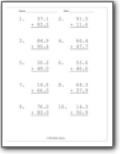Students will focus on working with all the major place values including: tenths, hundredths thousandths.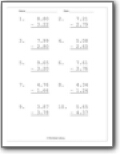###### Decimal Subtraction

Students will learn how to find the difference between two decimal values.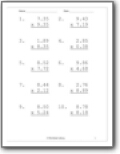###### Decimal Multiplication

Learn how to find the product of two decimals. You will need to start learning about the place holder of place values and how to keep track.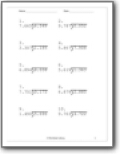###### Decimal Division

This is the operations that trips most students up with this form of values.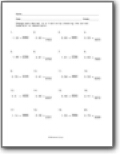###### Converting Between Decimals and Fractions

Students learn how the two systems complement one another and how to make simple conversions between them as needed.